UnboundEd Mathematics Guide

# Ratios: Unbound A Guide to Grade 6 Mathematics Standards

?
What's in a Content Guide and how do I use it?
Get answers to all your Content Guide questions, including what's in each part and how they can be used in your role at your school
View FAQs

6.RP.A | Understand ratio concepts and use ratio reasoning to solve problems.

Welcome to the UnboundEd Mathematics Guide series! These guides are designed to explain what new, high standards for mathematics say about what students should learn in each grade, and what they mean for curriculum and instruction. This guide, the first for Grade 6, includes three parts. The first part gives a “tour” of the standards for Ratios & Proportional Relationships using freely available online resources that you can use or adapt for your class. The second part shows how Ratios & Proportional Relationships relate to other concepts in Grade 6. And the third part explains where Ratios & Proportional Relationships are situated in the progression of learning in Grades 3-8. Throughout all of our guides, we include a large number of sample math problems. We strongly suggest tackling these problems yourself to help best understand the methods and strategies we’re covering, and the potential challenges your students might face.

# Part 1: What do these standards say?

Another reason that ratios are a great way to start the year is that they’re a “fresh” idea, introduced for the first time in Grade 6, and are accessible to any student with a basic understanding of whole-number multiplication. Moveover, some of the other work in Grade 6 (such as the standards for Expressions & Equations) is easier for students to understand once they have an understanding of ratios. So if you’re wondering where to start your year, ratios are a solid bet.

In Grade 6, the standards in the Ratios & Proportional Relationships (RP) domain are grouped together into one cluster (called RP.A because it’s the first and only cluster). But this one cluster contains a number of essential mathematical ideas. Let’s take a look at what these standards say.

6.RP.A Understand ratio concepts and use ratio reasoning to solve problems.

 6.RP.A.1 Understand the concept of a ratio and use ratio language to describe a ratio relationship between two quantities. For example, "The ratio of wings to beaks in the bird house at the zoo was 2:1, because for every 2 wings there was 1 beak." "For every vote candidate A received, candidate C received nearly three votes." 6.RP.A.2 Understand the concept of a unit rate a/b associated with a ratio a:b with b ≠ 0, and use rate language in the context of a ratio relationship. For example, "This recipe has a ratio of 3 cups of flour to 4 cups of sugar, so there is 3/4 cup of flour for each cup of sugar." "We paid \$75 for 15 hamburgers, which is a rate of \$5 per hamburger." * 6.RP.A.3 Use ratio and rate reasoning to solve real-world and mathematical problems, e.g., by reasoning about tables of equivalent ratios, tape diagrams, double number line diagrams or equations. 6.RP.A.3.A Make tables of equivalent ratios relating quantities with whole-number measurements, find missing values in the tables and plot the pairs of values on the coordinate plane. Use tables to compare ratios. 6.RP.A.3.B Solve unit rate problems including those involving unit pricing and constant speed. For example, if it took 7 hours to mow 4 lawns, then at that rate, how many lawns could be mowed in 35 hours? At what rate were lawns being mowed? 6.RP.A.3.C Find a percent of a quantity as a rate per 100 (e.g., 30% of a quantity means 30/100 times the quantity); solve problems involving finding the whole, given a part and the percent. 6.RP.A.3.D Use ratio reasoning to convert measurement units; manipulate and transform units appropriately when multiplying or dividing quantities. *Expectations for unit rates in this grade are limited to non-complex fractions.

The order of the standards doesn’t indicate the order in which they have to be taught. Standards are only a set of expectations for what students should know and be able to do by the end of each year; they don’t prescribe an exact sequence or curriculum. In this case, though, they are lined up pretty well: The first two standards name important conceptual understandings that students should have about ratios and rates, and the third says that students should be able to use these understandings to solve problems. It even goes on to give examples of the kinds of problems that students should encounter, as well as the types of strategies they should use. If you’re planning a unit on ratios, it makes sense to follow a similar sequence: Lay a conceptual foundation first, using simple problems, and then move on to more advanced applications of those ideas. And remember that instruction on ratios is probably going to involve working with more than one standard at once, since new concepts can often be introduced through real-world contexts and problems.

## Ratios and rates: Essential concepts

As adults, we do ratio math all the time without naming the ideas involved. But teaching students who are new to ratios requires precise knowledge of what they’re being expected to learn. Let’s pause for a moment to think about the concepts in these standards:

• A ratio is a pair of non-negative numbers, A:B, which are not both 0 (such as 1:2, 5:3, etc.).2 Much of the information in this section is taken from the Draft 6-7 Progression on Ratios and Proportional Relationships, one of a series of papers that describes the big ideas behind the standards and how those ideas fit together. If you’re interested in learning more about ratios and proportional relationships, it’s a good resource. Much of the information in this section is taken from the Draft 6-7 Progression on Ratios and Proportional Relationships, one of a series of papers that describes the big ideas behind the standards and how those ideas fit together. If you’re interested in learning more about ratios and proportional relationships, it’s a good resource. Much of the information in this section is taken from the Draft 6-7 Progression on Ratios and Proportional Relationships, one of a series of papers that describes the big ideas behind the standards and how those ideas fit together. If you’re interested in learning more about ratios and proportional relationships, it’s a good resource. Any two quantities can be associated in a ratio. Some examples of ratios which associate two quantities in this way are:

• I paid 1 dollar for every 4 pounds of flour. The ratio of dollars to pounds of flour is 1:4.
• For every 3 girls in the class, there are 2 boys. The ratio of girls to boys is 3:2.
• In the birdhouse at the zoo, the ratio of wings to beaks is 2:1.

• Ratios are most useful in situations which other, equivalent ratios have meaning. Equivalent ratios are the ratios obtained by multiplying the numbers in a ratio by the same positive number. So, for a class where the ratio of girls to boys is 3:2, we can generate equivalent ratios of 6:4, 9:6, 12:8, and so on. In context, this means that the class might have 6 girls and 4 boys, 9 girls and 6 boys, 12 girls and 8 boys, and so on. The number of students and the size of each group of boys and girls might change, but the ratios 3:2, 6:4, 9:6, and 12:8 are all equivalent. All of these are “in the same ratio.”

• The standards also focus on ratio language. In our last example, if the ratio of girls to boys is 3:2, we can say, “For every 3 girls there are 2 boys.” The phrase “for every” (and also “for each”) is an important piece of language that can be used to describe a ratio relationship.3 While “for every” will eventually take on a more widely applicable meaning in higher level math, in Grade 6, when used in a context associating two quantities, it is an indicator of a ratio relationship. While “for every” will eventually take on a more widely applicable meaning in higher level math, in Grade 6, when used in a context associating two quantities, it is an indicator of a ratio relationship. While “for every” will eventually take on a more widely applicable meaning in higher level math, in Grade 6, when used in a context associating two quantities, it is an indicator of a ratio relationship.

• Ratios have companion unit rates. A unit rate of two quantities in a ratio is the number of units of the first quantity for every 1 unit of the second quantity. (This is what standard 6.RP.A.2 means when it says “a unit rate a/b associated with a ratio a:b.”) For example, each of these statements contains a ratio followed by its associated unit rate:

• A recipe uses 1 cup of sugar for every 2 cups of flour, so there is 1/2 cup of sugar for every cup of flour.
• Juice costs 96 cents for every 32 ounces, which is a rate of 3 cents for each ounce.
• A car travels 120 miles every 2 hours, which is a rate of 60 miles per hour.

Note that in Grade 6, students only need to work with non-complex fractions (fractions of two whole numbers, not fractions of fractions). Starting in Grade 7, they’ll work with rates such as “1/2 mile for every 1/4 hour.” More on that in Part 3 of this guide.

The importance of conceptual understanding

Both standards 6.RP.A.1 and 6.RP.A.2 seem to focus on the language of ratio and rates. This is certainly an important aspect of these standards and deserves explicit attention. But at the heart of both standards is really the understanding of the concepts of ratio and unit rate, which will help students make sense of a variety of problems. (Remember, the cluster heading for these standards is, “Understand ratio concepts and use ratio reasoning to solve problems.”) As you plan lessons for these standards, think about how students can explain and show their understanding of ratios and rates in various ways. Ultimately, students should be able to define the concepts named above in their own words, as well as give examples and nonexamples of each.

## Problems that develop conceptual understanding

Now that those we’ve established the basics, the natural question is: How do we get students to start thinking in terms of ratios and rates? Let’s take a look at three tasks that could be used as part of a unit to develop student understanding of these concepts. These aren’t the only tasks you’ll need to teach ratios, but they should give you a starting point for some of your lessons.

### Ratio language and notation

The task in the box below presents opportunities for students to use ratio language, to examine how ratios are distinct from other types of relationships, and to relate ratio statements to their numerical representations. (6.RP.A.1) It could be the basis for an early lesson on ratio language and notation.

#### Games at Recess

The students in Mr. Hill’s class played games at recess.

6 boys played soccer

4 girls played soccer

2 boys jumped rope

8 girls jumped rope

Afterward, Mr. Hill asked the students to compare the boys and girls playing different games.

Mika said,

“Four more girls jumped rope than played soccer.”

“For every girl that played soccer, two girls jumped rope.”

Mr. Hill said, “Mika compared the girls by looking at the difference and Chaska compared the girls using a ratio.”

1. Compare the number of boys who played soccer and jumped rope using the difference. Write your answer as a sentence as Mika did.

1. Compare the number of boys who played soccer and jumped rope using a ratio. Write your answer as a sentence as Chaska did.

1. Compare the number of girls who played soccer to the number of boys who played soccer using a ratio. Write your answer as a sentence as Chaska did.

HideShow

One interesting aspect of this task is how it contrasts ratio language with the language of difference, so that students begin to see how related quantities can be described in more than one way. Though this might seem obvious, it’s a good way to draw students’ attention to the fact that ratio relationships are distinct from the types of additive comparisons (“how many more” and “how many less”) that they may have seen in the elementary grades. Moreover, by contrasting their answers to parts (b) and (c), they’re able to see that situations often give rise to a number of possible ratio relationships. Since the directions for this task are fairly simple, you might also use it as an opportunity to explicitly teach ratio notation (e.g., 1:2). Or, if you’re using this task after your students have learned ratio notation, having them express each statement numerically could be a way to practice. You could even ask them to write several more ratio statements based on the information in the task to emphasize the various ratios in the problem.

### Equivalent ratios

Soon after students start working with ratios, they should begin to see how one ratio implies a set of other, equivalent ratios. Ratio tables make it easy for students to see relationships among ratios: they present both an additive structure and a multiplicative structure, and students should be familiar with both. The task in the box below is taken from a lesson that does just that, encouraging students to discover and use more than one relationship in a table.

#### Grade 6, Module 1, Lesson 10: Exit Ticket

Show more than one way you could use the structure of the table to find the unknown value. Fill in the unknown values.

 Number of Weeks Amount of Money in Account 2 \$350 4 \$700 6 \$1,050 8 10

When using this task (or one like it), it’s important to observe the ways in which they think about finding unknown values. Ask students to describe how they determined the amount of money in each row. Students who are able to see the multiplicative structure will be able to say things like, “I could see that each value in the second column is 175 times the corresponding value in the first column.” Students who are able to see the additive structure might say things like, “I could see that the values in the first column increase by two and the values in the second column increase by 350.” Though both observations are valid, it’s important that students see the multiplicative structure between quantities to get ready for questions like, “How much money would be left in the account after 26 weeks?” In that case, a student could find the answer by extending the table and continuing to add, but it’s far more efficient to multiply. If some students don’t see the multiplicative relationship at first, you (or another student) could illustrate it on the board, using arrows to show the patterns within the table. You might also choose to extend this task by adding another row of the table with a more challenging number of weeks (such as 11 or 26) to help students understand why the multiplicative structure is so valuable.

### Unit rates

Unit rates are a natural extension of ratios. (6.RP.A.2) The task below is interesting because it uses unusual items to get students thinking about the notion of unit rate as “for every” or “per 1” of a second quantity, and to reinforce the importance of order in expressing rates.

#### Hippos Love Pumpkins

1. Hippos sometimes get to eat pumpkins as a special treat. If 3 hippos eat 5 pumpkins, how many pumpkins per hippo is that?1. Lindy made 24 jelly-bread sandwiches with a 16-ounce jar of jelly. How many ounces of jelly per sandwich is that?

1. Purslane bought 350 rolls of toilet paper for the whole year. How many rolls of toilet paper per month is that?

1. In the world's longest running experiment, scientists have tried to capture tar pitch dripping on camera. In the past 86 years, 9 drops have formed. How many years per drop is that?

1. Imagine that 12 goats got into a dumpster behind a pizza parlor and ate 3 pizzas. How many goats per pizza would that be?

HideShow

One way to extend this task might be to ask students to find two distinct rates in each situation (such as “goats per pizza” and “pizzas per goat”), or to ask why expressing a rate in a certain way might be useful (if a zookeeper is deciding how many pumpkins to buy for 8 hippos, for example). You could also ask some simple questions involving rate reasoning. (6.RP.A.3.B) (For example, “If Lindy makes 3 more sandwiches, how much jelly will she need?”) On the other hand, if you think your students might not be ready for the complexity of the numbers involved here, consider adding a couple of examples with very “clean” unit rates up front. Any situation with a whole-number unit rate would be fine (“Tanasia watched 3 shows and saw 18 commercials. How many commercials per show is that?”), as would a situation where the unit rate is a simple fraction like 1/2 (“Doug can eat 8 burgers in 4 minutes. How many minutes per burger is that?”).

There are four specific representations named in these standards that students can use to better understand and solve problems with ratios and rates: tape diagrams, ratio tables, double number lines, and equations. Each of them is described below, but it’s a good idea to try them all yourself before using them in your lessons. You’ll probably find that each has strengths and limitations. It’s also important to know that students will need explicit instruction on why and how to use each type of representation. Introduce tape diagrams and double number lines early, when your students are working with simple problems. That way, they’ll have time to get to know these representations, and will be able to use them more effectively when problems become more challenging. Over time, they should see how the right tool can make complicated problems more manageable.

Why not just use cross multiplication?

Most adults have seen some version of the “cross multiplication” method of solving ratio problems, which involves writing equations like these and multiplying on the diagonal: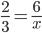This method relies on a multi-step algorithm that students often don’t understand, and they use it without realizing why it works. (In the equation above, why does multiplying 6 by 3, and then dividing by 2 yield the correct answer? Most students would have a hard time explaining.) When students don’t understand why a procedure works, it becomes more likely that they will misapply it. Though equations like this can be useful for solving ratio problems, the cross multiplication method isn’t required in Grade 6 for exactly this reason. The standards require students to build deep conceptual understanding of ratios and rate, which they can then use to solve problems. Over time, they should be able to use a variety of representations and methods to explain their thinking, rather than relying on a single method.

### Tape diagrams

Tape diagrams (also known as bar models or strip diagrams) are thin rectangles resembling pieces of tape that can be divided into sections to represent parts of a problem. In the elementary grades, a tape diagram with two sections can represent a simple addition or subtraction problem; later on, a diagram with several equal-sized sections can represent a multiplication or division problem. Students can represent ratio problems using two tape diagrams “side by side” to represent the two quantities in a ratio relationship. Because they offer a handy way to visualize the elements of a problem, tape diagrams will probably be among the first tools you’ll use with students. They also help to build conceptual understanding of equivalent ratios, as in this task.

#### Grade 6, Module 1, Lesson 3: Exercise 2

Shanni and Mel are using a ribbon to decorate a project in their art class. The ratio of the length of Shanni’s ribbon to the length of Mel’s ribbon is 7:3.

Draw a tape diagram to represent this ratio.HideShow

When used early in the unit on ratios, this task can help students understand the nature of equivalent ratios. Since each model has the same number of units, students can see that equivalent ratios all have the same unit rate. (This is one of the defining features of equivalent ratios.) You might push students to explain this concept by asking what all three ratios have in common, and then asking them to find another ratio that’s equivalent to the others (and prove its equivalence by using a model).

Tape diagrams are also useful aids for problem-solving. The process of constructing a tape diagram from a problem requires students to read the problem, determine the quantities involved, and determine the relationships among those quantities. Once they’re able to represent the problem in diagram form, they should have a solid grasp of what operations they need to use to solve it. At first, students might find it tedious to create a diagram and then find a solution to the problem, but tape diagrams have two advantages. First, they help students slow down and think about each problem before they try just anything. Second, they allow students to decode very tricky problems that are difficult to solve using only an arithmetic method. Take this problem, for example.

#### Grade 6, Module 1, Lesson 6: Exercise 1

The Business Direct Hotel caters to people who travel for different types of business trips. On Saturday night there is not a lot of business travel, so the ratio of the number of occupied rooms to the number of unoccupied rooms is 2: 5. However, on Sunday night the ratio of the number of occupied rooms to the number of unoccupied rooms is 6: 1 due to the number of business people attending a large conference in the area. If the Business Direct Hotel has 432 occupied rooms on Sunday night, how many unoccupied rooms does it have on Saturday night?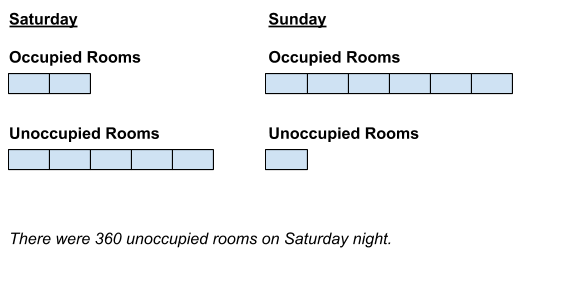HideShow

Without drawing the diagrams first, the relationships between the two ratios (2:5 and 6:1) and the given quantity (432 occupied rooms on Sunday) might be tough to discern. But once students take the time to construct both tape diagrams, the situations becomes much more clear. Finding the solution just requires a few simple calculations, as shown here:

6 units = 432 rooms

1 unit = 432 ÷ 6 = 72 rooms

5 units = 72 × 5 = 360 rooms

### Ratio tables

In addition to being a useful instructional tool, ratio tables are also useful for solving problems. They’re simple to construct, help students organize their work, and are adaptable to a variety of situations. This task is just one example.

#### Mixing Concrete

A mixture of concrete is made up of sand and cement in a ratio of 5:3. How many cubic feet of each are needed to make 160 cubic feet of concrete mix?

While students might represent this problem in a number of ways, a ratio table might be the fastest and simplest: They can easily record the facts given in the problem, as well as the quantities they need to find. The table below includes two extra columns, which aren’t required to solve the problem but might help struggling students. Asking them to explain how to complete these intermediate rows might give them a clue about how to get started.

 Sand 5 10 ? Cement 3 9 ? Total Mixture 8 16 160

### Double number lines

Just as the name implies, double number lines are diagrams containing two parallel number lines labeled with different units. They’re similar to ratio tables in that they allow students to easily scale quantities up or down, but also allow students to see the relationship between different units as in a tape diagram. (In particular, double number lines are handy where percents are involved, allowing students to make sense of problems beyond those that just require finding percent of a number.) The task below relates ounces of soda and grams of sugar, allowing students to solve based on a ratio of 20 grams of sugar to every 6 ounces of soda.

#### Grade 6, Module 1, Lesson 12: Exercise 4

A school cafeteria has a restriction on the amount of sugary drinks available to students. Drinks may not have more than of sugar. Based on this restriction, what is the largest size cola (in ounces) the cafeteria can offer to students?My estimate is between 6 and 12 oz but closer to 6 ounces. I need to find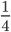of 6 and add it to 6.x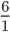=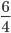= 1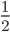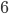+ 1= 7A 7oz cola is the largest size that the school cafeteria can offer to students.

HideShow

Notice how the double number line allows students to see where the answer falls in a range of equivalent ratios. The calculations shown are only one possible solution; students could also use unit rate reasoning, for example, to find the amount of soda per gram of sugar, and then multiply to find the amount of soda containing 25 grams of sugar. (If given a choice of methods, students will probably find yet more ways to solve.)

### Equations

After students have had plenty of exposure to conceptual representations like ratio tables and tape diagrams, they may be ready to solve certain problems by writing equations. The “Mixing Concrete” problem above, for example, could be solved with equations. Notice, however, how closely these equations resemble portions of the table that students used to approach the problem at first. Learning to write equations like this should be the natural result of working with ratio tables, so that after enough “at bats,” students are able to explain how their equations are valid expressions of the problem situation.

 Sand 5 Cement 3 Total Mixture 8 160

 Sand 5 Total Mixture 8 160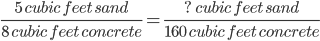Cement 3 Total Mixture 8 160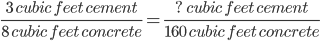It’s also important to note that when students “solve” these equations, they shouldn’t need to use abstract methods like “cross multiplication.” Rather, they’ll discover the unknown factor that relates two of the quantities in the problem and use that to calculate the answer. This way, they don’t have to employ any “tricks,” but can rely on established ideas about equivalent ratios and the relationship between multiplication and division.

8 cubic feet concrete × __ = 160 cubic feet concrete

8 cubic feet concrete × 20 = 160 cubic feet concrete

5 cubic feet sand × 20 = 100 cubic feet sand

## Applications of ratios and rates

Once the concept of ratio has been introduced, students should get plenty of practice solving a variety of ratio problems. A balanced instructional unit will include problems that involve finding equivalent ratios and also problems that require students to work with ratio tables and graph ratio relationships on the coordinate plane. (6.RP.A.3.A) This example involves both: Students need to complete a ratio table based on a description of a situation, and then use their entries in the table to create a graph.

#### Grade 6, Module 1, Lesson 14: Example 1

Kelli is traveling by train with her soccer team from Yonkers, NY to Morgantown, WV for a tournament. The distance between Yonkers and Morgantown is 400 miles. The total trip will take 8 hours. Dinner service starts once the train is 250 miles away from Yonkers. What is the minimum time the players will have to wait before they can have their meal?The minimum time is 5 hours.

HideShow

The questions that follow this problem (in the linked document) are interesting, first in how they explicitly help students set up the graph (students may require more or less guidance than this lesson plan suggests, but it’s good to think about how they’ll interact with the coordinate plane, which was only just introduced in Grade 5). Then, when looking at the completed graph, ask students to interpret the meaning of individual points (“What does this point represent in the context of distance and time?”). Constantly considering how the parts of a representation relate to the original situation is a good habit of mathematical thinking to encourage. It’s also worth pointing out that this lesson plan budgets quite a bit of time for this problem, allowing students to fully engage with all of its aspects.

In addition to using equivalent ratios to solve problems, students should also be able to use unit rate thinking to solve problems. This task invites multiple solutions: students can set up a ratio table and “jump” to the values they need for each part, but they can also find a unit rate.

#### Running at a Constant Speed

A runner ran 20 miles in 150 minutes. If she runs at that speed,

1. How long would it take her to run 6 miles?
2. How far could she run in 15 minutes?
3. How fast is she running in miles per hour?
4. What is her pace in minutes per mile?

If this task is used in the course of a lesson and students are allowed to solve in several ways, it might be interesting to discuss the contrasts among the methods they used with the class. How are they similar and different, in terms of the operations involved? Which one is the most efficient? Ultimately, students should be comfortable with both methods--there will be times when thinking in terms of unit rates is preferable to thinking in terms of equivalent ratios, especially when the numbers involved are less “friendly” than the ones used here—and seeing the connection between the two is valuable. If we want to encourage students to try one method over another, consider the order of the questions: As they’re presented now, students may be inclined to set up a ratio table to answers parts (a) and (b). But if parts (c) and (d) were moved first, they might be more inclined to use a unit rate solution.

One remarkable aspect of the ratio standards is that percents and measurement conversions, which in the past were often taught as isolated skills, are now treated as ratio situations. This is good news, as students are now able to approach them using the same type of reasoning and tools as with other ratio problems. This task is a fairly straightforward percent problem.

#### Kendall’s Vase

Kendall bought a vase that was priced at \$450. In addition, she had to pay 3% sales tax. How much did she pay for the vase?

One of the sample solutions shows a percent table (really, a type of ratio table) that illustrates the relationship between ratios and percents. Double number lines (like those shown in the section above) could also help students find the correct answer. Similar strategies can be employed to solve problems involving measurement conversions.

## The role of Mathematical Practices

The standards don’t just include knowledge and skills; they also recognize the need for students to engage in certain important practices of mathematical thinking and communication. These “Mathematical Practices” have their own set of standards, which contain the same basic objectives for Grades K-12.4 You can read the full text of the Standards for Mathematical Practice here. You can read the full text of the Standards for Mathematical Practice here. You can read the full text of the Standards for Mathematical Practice here. (The idea is that students should cultivate the same habits of mind in increasingly sophisticated ways over the years.) But rather than being “just another thing” for teachers to incorporate into their classes, the practices are ways to help students arrive at the deep conceptual understandings required in each grade. In other words, the Practices help students get the content. The table below contains a few examples of how the Mathematical Practices might help students understand and work with ratios in Grade 6.

 Opportunities for Mathematical Practices: Teacher actions: Percents are a special kind of rate (a “rate per 100”) which is similar in structure to unit rate (a “rate per 1”). When students are allowed to notice and explain this concept, and then to apply it in a variety of problem contexts, they look for and make use of structure. (MP.7) Consider introducing percents by showing students examples in which it helps to compare several quantities on a “per 100” basis. (For an example, see Exercises 1-2 of this introductory lesson plan on percents). Ask them to explain how this approach is similar and different to using a unit rate. And when students are able to use the “per 100” structure of percents to solve problems (e.g. they figure out that a 25-cup recipe with 7 cups of sugar contains 28% sugar using multiplication by 4), you can have them explain their reasoning to the rest of the class. Students can model with mathematics (MP.4) when they solve real-world and mathematical problems using ratio and rate reasoning, especially when they make use of various representations in the modeling process. Show students a variety of ways to model ratio situations (such as those presented above: tape diagrams, ratio tables, double number lines, and equations), and during the course of the year, give them interesting problem contexts that require these models. Have students explain how their representations model the situation at hand, and why they selected a certain type of representation for a given situation. Students can reason abstractly and quantitatively (MP.2) when they work with the concept of a ratio, which abstracts a comparison between two quantities and implies other, related relationships. (For example, a ratio of 3:4 implies equivalent ratios of 6:8, 9:12, etc., and a unit rate of 3/4.) As a simple exploration activity, you might have students count intentionally constructed sets of objects—like checkers—where there is a consistent ratio of black checkers to red. To start, students are simply counting (quantifying) what they observe. Then you can guide students to look for patterns between the numbers of black and red checkers in each group. After examining several groups—perhaps one group per table of students—students should come to see the underlying structure of a black checkers to b red checkers. Finally, you can abstract this structure using ratio language (e.g. “For every a black checkers, there are b red checkers.”).

Podcast clip: Importance of the Mathematical Practices with Andrew Chen and Peter Coe (start 30:33, end 43:39)

# Part 2: How do ratios relate to other parts of Grade 6?

There are lots of connections among standards in Grade 6; if you think about the standards long enough, you’ll probably start to see these relationships everywhere.5 The idea that standards relate strongly to one another is known as coherence, and is a distinctive feature of the Common Core State Standards for Mathematics. If you’re interested in exploring more of the connections between standards, you might want to try the Student Achievement Partners Coherence Map web app. The idea that standards relate strongly to one another is known as coherence, and is a distinctive feature of the Common Core State Standards for Mathematics. If you’re interested in exploring more of the connections between standards, you might want to try the Student Achievement Partners Coherence Map web app. The idea that standards relate strongly to one another is known as coherence, and is a distinctive feature of the Common Core State Standards for Mathematics. If you’re interested in exploring more of the connections between standards, you might want to try the Student Achievement Partners Coherence Map web app. A few are so important, though, that they deserve special attention. The first is the relationship that the ratio and proportional reasoning standards have to one another. As mentioned above, it’s tough to work in one of these standards without working in others at the same time. When students are starting to understand the concepts of ratio and unit rate, (6.RP.A.1, 6.RP.A.2) they should be solving application problems that draw out these ideas. (6.RP.A.3) Exploring problems through ratio tables, for example, is a great way to see how ratio relationships work. And as students begin solving a wider variety of problems (rate problems, percent problems, measurement conversions), they’ll be cementing their core understandings of ratio and rate through application. Therefore, as you’re planning instruction for one standard, keep the others in mind: There may be clues about how to teach one standard hidden in another.

## Expressions & Equations: Reasoning about one-variable equations

Another area of focus in Grade 6 is work with algebraic Expressions & Equations (EE). This is an area that deserves its own guide, but right now we’ll cover two particular ways that ratios can play a part in understanding these concepts. Many teachers will choose to begin their study of EE with standards that relate expressions to arithmetic operations from Grades K-5. (6.EE.A.2) When it comes time to write and solve equations for a variety of situations, (6.EE.B.7) though, ratio situations are a rich source of problems, and ratio reasoning can lend students insight into how to write equations to model a situation. This task is one such example:

#### Firefighter Allocation

A town's total allocation for firefighter's wages and beneﬁts in a new budget is \$600,000. If wages are calculated at \$40,000 per firefighter and beneﬁts at \$20,000 per firefighter, write an equation whose solution is the number of firefighters the town can employ if they spend their whole budget. Solve the equation.

The commentary and solutions that follow the task explain how to set up an equation to solve this problem. But writing expressions and equations is very abstract, and can be tough for many students when they try it the first time around. So how do students get to the point where they can write equations like the ones suggested? One way to get started is to help students see a ratio at work in this problem (for every firefighter it hires, the town spends \$60,000) and explore it using a ratio table. You might guide students to build a table like the one below by asking:

• How much is the town spending per firefighter?
• How much for two firefighters? How do you know?
• What is the relationship between the first and second row?

 Firefighters 1 2 3 x Cost (\$) 60,000 120,000 180,000

By applying the same type of unit rate reasoning they used to solve ratio problems, students can see why a good expression for the total cost of x firefighters is 60,000x. Some questions you might want to ask to help your students come to this realization are:

• What does the x represent? What does the box below x represent?
• What would the cost for 4, 5, and 6 firefighters be? How do you know?
• Can you write some expressions that show how to find the cost in each column of the table? What changes from one expression to the next, and what stays the same? How could these help you fill in the last box?

Solving problems like this will help to prepare students for more work with algebraic equations and ratios in Grade 7. (7.RP.A.2.C)

600,000 = 60,000x

↑ unit rate

Since this standard also requires that students work with equations involving additive relationships (equations of the form x + p = q), students should also learn to distinguish situations where two quantities are in a ratio relationship and when they’re not. A table may also help when introducing the difference between the two situations, as it allows students to easily see when there is no multiplicative relationship between rows.

## Expressions & Equations: Representing relationships between two variables

In addition to writing and solving equations with one variable (like the one shown above), students also begin using equations in two variables to model the relationship between two quantities. (6.EE.C.9) This is where their journey toward modeling with functions, which continues through the rest of the middle grades and into high school, really begins. Many of the situations that they encounter will involve ratios and rates, so this is where prior experience with those concepts will come in handy. As an example, let’s look at this task:

#### Chocolate Bar Sales

Stephanie is helping her band collect money to fund a field trip. The band decided to sell boxes of chocolate bars. Each bar sells for \$1.50 and each box contains 20 bars. Below is a partial table of monies collected for different numbers of boxes sold.

 Boxes SoldMoney Collected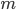1 \$30.00 2 3 4 5 \$150.00 6 7 8

1. Complete the table above for the values of.

1. Write an equation for the amount of money,, that will be collected ifboxes of chocolate bars are sold. Which is the independent variable and which is the dependent variable?

1. Graph the equation using the ordered pairs from the table above.

1. Calculate how much money will be collected if 100 boxes of chocolate bars are sold.

1. The band collected \$1530.00 from chocolate bar sales. How many boxes did they sell?

HideShow

Many parts of this problem should be familiar to students after studying ratios and rates: They’re making a table to represent the constant relationship between boxes and money, they’re graphing that relationship on the coordinate plane, and they’re using a rate to find unknown quantities.

Part (b) is the tricky one, though, so let’s focus on that. The difficulty here is the same as in the previous task: Writing equations is very abstract, and students often have trouble seeing and expressing the relationship between variables. Not surprisingly, the questions you might ask to help students write the equation are also similar. (And fortunately, this problem has a table built into it, which can help students see the relationships involved.) You might ask:

• What is the cost per box? How do you know?
• What relationship do you see between columns in the table?
• Can you write some expressions to show how to find the cost in each row of the table? What changes from one expression to the next, and what stays the same? What if the number of boxes is b?

Again, as students solve more problems like this, they’ll begin to see that they can model any two variables in a ratio relationship in the same way:

dependent variable ↓ ↓ independent variable

m = 30b

↑ unit rate

This isn’t limited to quantities in a ratio relationship. Students should also see situations where there is an additive relationship between variables (e.g., x + 5 = y). Again, tables are helpful in allowing students to see that no multiplicative relationship exists in these cases.

## The Number System: Operations with decimals

The last significant connection that we want to mention here is the relationship to operations with decimals. (6.NS.B.2, 6.NS.B.3) Because working fluently with multidigit decimals is a required fluency for Grade 6, students should be given frequent opportunities to use all four standard algorithms with decimals. As mentioned above, ratio and rate work is a good place to start the year; study of the standards in the Number System (NS) domain will likely occur later on in the year. However, once instruction on dividing multi-digit whole numbers (6.NS.B.2) and the four operations with multidigit decimals (6.NS.B.3) has occurred, ratio and rate problems are good ways to practice these skills and develop fluency while continuing to deepen their understanding of ratio. Take this problem, which invites students to use multiple algorithms:

When making bread, a baker uses 2 ounces of yeast for every 8 pounds of flour. How much yeast does the baker need to make a batch of bread using 150 pounds of flour?

Though there are a number of ways to solve this, one approach would be to calculate a unit rate (0.25 ounces of yeast per pound of flour) using the long division algorithm, and then multiply (again, using the standard algorithm) by the amount of flour in the larger batch to find the amount of yeast required. Students might interpret the problem using ratio reasoning and a representation like a ratio table or tape diagram, and then build fluency with decimal operations in calculating the solution.

# Part 3: Where do ratios come from, and where are they going?

Podcast clip: Importance of Coherence with Andrew Chen and Peter Coe (start 9:34, end 26:19)

## Where do ratios come from?

### Grades 3-5: Multiplication and division

Starting in Grade 3, students are introduced to multiplication (and, in a related way, division) as an expression of equal groups. (3.OA.A.1, 3.OA.A.2) In Grade 4, they should begin to understand the idea of multiplicative comparison (the idea that one quantity is so many times more than another) and distinguish this from additive comparison (the idea that one quantity is simply so many more than another). (4.OA.A.1, 4.OA.A.2) The differences between these concepts are shown below:

 Multiplicative comparison “I have 2 apples and Samantha has 10 apples. How many times more does Samantha have than I do?” 2 × ___ = 10 Additive comparison “I have 2 apples and Niyah has 10 apples. How many more apples does Niyah have than I do?” 2 + ___ = 10

In Grade 5, students should expand their concept of multiplication again to interpret multiplication as scaling. (5.NF.B.5) This occurs in the context of work with fractions, but as in Grade 4, the focus is still on how two quantities are related by an unknown factor. In both Grades 4 and 5, reasoning about multiplication strongly resembles later thinking around ratios. When we line up a few problems from each grade, we can see students making their way toward ratios through the years.

 Grade 3 Liam bought 5 bunches of bananas. Each bunch has exactly 5 bananas. How many bananas does Liam have? Mrs. Oro needs 90 corn seeds. The Garden Center sells corn seeds in packs of 10 seeds each. Write a division equation showing how many packs of seeds Mrs. Oro should buy. (Source: “Introducing Multiplication and Division Unit Plan” by Student Achievement Partners is licensed under CC 0 1.0.) ➔ With multiplication and division problems like these, students use the ideas of multiplication as equal groups and division as an unknown factor. These are the foundation for all future work involving multiplication and division, including ratios.

 Grade 4 The Turner family uses 548 liters of water per day. The Hill family uses 3 times as much water per day. How much water does the Hill family use per day? How much water will they use per week? (Source: Grade 4, Module 3, Lesson 12 (teacher version) from EngageNY.org of the New York State Education Department is licensed under CC BY-NC-SA 3.0.) ➔ With multiplicative comparison problems like this one, students begin to reason about how two numbers might be related by a certain factor. They’ll use similar reasoning to scale numbers up and down by fractional factors in Grade 5 and to find equivalent ratios in Grade 6.

 Grade 3 Use the fractional units on the left to count up on the number line. Label the missing fractions on the blanks.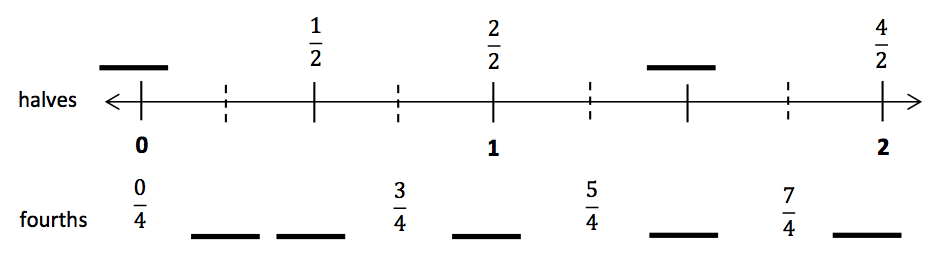(Source: Grade 3, Module 5, Lesson 21 (teacher version) from EngageNY.org of the New York State Education Department is licensed under CC BY-NC-SA 3.0.) ➔ As they begin work with fractions, students use the number line (along with other representations) to show how fractions are composed of unit fractions. (In this diagram, for example, they can see that 3/4 is composed of 3 intervals that are each 1/4 long.) They also begin to understand the ideas behind fraction equivalence by dividing the number line in different ways.

 Grade 4 Draw a number bond and write a number sentence to match the tape diagram.(Source: Grade 4, Module 5, Lesson 1 (teacher version) from EngageNY.org of the New York State Education Department is licensed under CC BY-NC-SA 3.0.) ➔ With problems like this, Grade 4 students learn to see fractions as sums of other fractions with the same unit size (in this example, 4/5 = 1/5 + 3/5). This will help them to develop methods for adding and subtracting fractions, and later, to understand multiplication of fractions by whole numbers.

 Grade 5 Carly and Gina read the following problem in their math class. Seven cereal bars were shared equally by 3 children. How much did each child receive? Carly and Gina solve the problem differently. Carly gives each child 2 whole cereal bars, and then divides the remaining cereal bar among the 3 children. Gina divides all the cereal bars into thirds and shares the thirds equally among the 3 children. a. Illustrate both girls’ solutions. b. Explain why they are both right. (Source: Grade 5, Module 4, Lesson 2 (teacher version) from EngageNY.org of the New York State Education Department is licensed under CC BY-NC-SA 3.0.) ➔ In this problem, students are asked to interpret the fraction 7/3 in two different ways. Notice the phrase “for each” in the question; this is basically a unit rate problem. Solving problems like this prepares students for a formal understanding of unit rates in Grade 6.

Students are also expected to work with arithmetic patterns in the elementary grades. In Grade 3, they look for and explain certain patterns in the multiplication table—noticing, for example, that every other number in the 2 sequence is a number in the 4 sequence. (3.OA.D.9) In Grade 4, this type of thinking should extend to other patterns outside of the multiplication table. (4.OA.C.5) And in Grade 5, they further their understanding by generating two arithmetic sequences, explaining the relationships between them, and plotting them on the coordinate plane. (5.OA.B.3) This sequence of problems shows how the standards build a foundation in number patterns that students can use when approaching ratios.

 Grade 3 The table shows products of the whole numbers 1 through 6.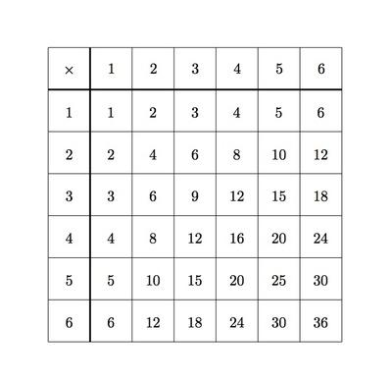(a) Color all of the even products in the table. (b) Sometimes there are even numbers next to each other in the table. However, there are never odd numbers next to each other. Why is this true? (Source: “Patterns in the Multiplication Table” by Illustrative Mathematics is licensed under CC BY 4.0.)

 Grade 4 a. Starting with 9, list the first 10 multiples of 9. b. In the list in part (a), what patterns do you see with the digits in the 10's place? What patterns do you see with the digits in the 1's place? c. Using pictures, words or equations, explain the patterns you observed in part (b). (Source: “Multiples of Nine” by Illustrative Mathematics is licensed under CC BY 4.0.)

 Grade 5 Create a table of 3 values for x and y such that each y-coordinate is 3 more than the corresponding x-coordinate.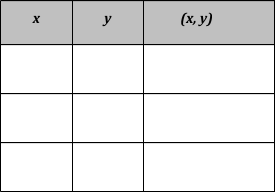(a) Plot each point on the coordinate plane.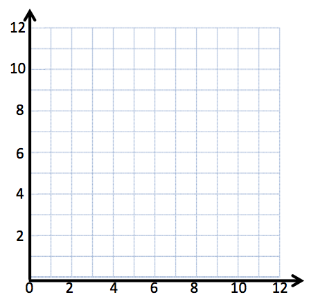(b) Use a straightedge to draw a line connecting these points. (c) Give the coordinates of 2 other points that fall on this line with x-coordinates greater than 12. Source: Grade 5, Module 6, Lesson 8 (teacher version) from EngageNY.org of the New York State Education Department is licensed under CC BY-NC-SA 3.0.

Ratios represent a capstone of this sequence, in that students are consolidating their ideas of multiplication, division and patterns into the concept of a ratio. When approaching ratios for the first time, it may help to present students with problems that result in two rows of a multiplication table. For example, a drink recipe requiring 2 cups of cranberry juice for every 5 cups of apple juice gives students two very familiar sequences, which can help them begin to understand ratios as multiplicative comparisons.

### Suggestions for students who are below grade level

• If a significant number of students don’t understand the notion of multiplicative comparison, you could plan a lesson or two on that idea before starting work with ratios. (This Grade 4 lesson, which includes several multiplicative comparison problems, might be helpful.) And if you think an entire lesson is too much, but your students could still use some review, you could create 2-3 multiplicative comparison problems as “warm-ups” to start your first few lessons on ratios.
• If a significant number of students don’t understand fractions as division, you could likewise plan a lesson or two on that before introducing rates. (This Grade 5 lesson, which involves some “for each” problems that lead nicely into unit rates, is one possible starting point.) Again, if you think that an entire lesson is too much, you could use some problems involving this idea as warm-ups for your first few rate lessons.
• If a significant number of students don’t understand the relationship between multiplication and division, you could plan a lesson or series of warm-ups on this idea. (This Grade 3 lesson offers some ideas of the types of models and problems you might use, and this Grade 4 lesson has some more advanced division problems that may be appropriate for older students.)
• If a significant number of students don’t understand the concept of equivalent fractions, or can’t fluently perform the procedures to find equivalent fractions, you could plan review lessons or a series of warm-ups on those topics. (This Grade 4 lesson might be a good place to start.)
• For students who lack fluency with multiplication and division facts, consider incorporating drills on these facts into your weekly routine. These can be fast-paced and joyful, and as students achieve improved fact fluency, they’ll be better able to perform the calculations involved in ratio problems. (For an example of this type of activity, see the instructions for a “sprint” on pages 11-13 of this document, as well as the sprint handout on pages 58-59 of this Grade 4 lesson plan.)

Podcast clip: Coherence with Ratios in Grade 6 with Andrew Chen and Peter Coe (start 26:20, end 30:31)

## Beyond Grade 6: What’s next with ratios?

### Grades 7-8: Proportional relationships, linear equations and functions

So how will students use their knowledge of ratios after Grade 6? This is an important question, because the answer defines the limits of instruction in Grade 6 and explains why it’s important to focus on a small number of essential concepts there. At the same time, knowing the next steps in the journey can help us focus our lessons on the knowledge and skills that matter most.

Let’s recap, then: By the end of Grade 6, students should have a firm grasp of the concepts of ratio and rate, and should have extensive experience with a variety of ratio problems (including percents and measurement conversions, which they should see through the lens of ratio reasoning).

From there, students move to Grade 7, where a few new things happen. First, ratio and rate problems get more challenging: Students will find unit rates of fractional quantities (7.RP.A.1) and will work on solving more complicated, multistep ratio and percent problems. (7.RP.A.3) Second, their concept of equivalent ratios will evolve into an understanding of proportional relationships, and students will explore these relationships through various representations. (7.RP.A.2.A) And third, the content the Ratios & Proportional Relationships and Expressions & Equations domains, which looked fairly distinct in Grade 6, will start to come together. (7.RP.A.2.C)

In Grade 8, there is no separate group of standards for ratios and proportional relationships; these ideas merge completely with the algebra content in the Expressions & Equations domain and the Functions domain. The concept of unit rate evolves into slope, (8.EE.B.5) and students will discover important properties of slope. They explore the connection between proportional relationships (i.e. can be represented by an equation y = mx) and linear equations more generally (y = mx + b). (8.EE.B.6) And after being formally introduced to the concept of a function, students will model linear relationships with functions. (8.F.B.4) Students will rely on these concepts throughout high school and, in many cases, in post-secondary work as well.

These are only brief summaries of the content in each grade, but hopefully they show how critical the learning in Grade 6 is to everything that follows. If students don’t have the time to develop deep conceptual understanding of ratios and unit rates in Grade 6, for example, the multistep problems that come in Grade 7 will be much more difficult. To highlight the limits of the expectations for each grade, as well as to show how each grade’s content depends on what came before, let’s take a look at one last progression of problems.

 Grade 7 Anthony’s mother is making birthday cupcakes for 12 friends at daycare. The recipe calls for 3 1/3 cups of flour. This recipe makes 2 1/2 dozen cupcakes. Anthony’s mother has only 1 cup of flour. Is there enough flour for each of his friends to get a cupcake? (Source: Grade 7, Module 1, Lesson 11 (teacher version) from EngageNY.org of the New York State Education Department is licensed under CC BY-NC-SA 3.0.) ➔ This problem illustrates the additional layer of computational difficulty in Grade 7 work with rates: Now students are working with complex fractions.

 Grade 8 The information in the table below shows the function of time in minutes with respect to mowing an area of lawn in square feet.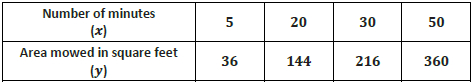Write a function rule that describes the area in square feet mowed, y, in x minutes. Then use your function rule to find the area of lawn that can be mowed in 24 minutes. (Source: Grade 8, Module 5, Lesson 3 (teacher version) from EngageNY.org of the New York State Education Department is licensed under CC BY-NC-SA 3.0.) ➔ This problem is representative of Grade 8 work with linear functions, which relies on understandings of proportional relationships from Grades 6 and 7. Here, students aren’t just finding a unit rate, but using that rate to build a function that models the situation.

Student Achievement Partners: Focus in Grade 6

Draft 6-7 Progression on Ratios and Proportional Relationships

EngageNY: Grade 6 Module 1 Materials

# Endnotes

 In this series, major clusters are denoted by a . For more information on the major work of Grade 6, see the Student Achievement Partners guide Focus in Grade 6.

 Much of the information in this section is taken from the Draft 6-7 Progression on Ratios and Proportional Relationships, one of a series of papers that describes the big ideas behind the standards and how those ideas fit together. If you’re interested in learning more about ratios and proportional relationships, it’s a good resource.

 While “for every” will eventually take on a more widely applicable meaning in higher level math, in Grade 6, when used in a context associating two quantities, it is an indicator of a ratio relationship.

 You can read the full text of the Standards for Mathematical Practice here.

 The idea that standards relate strongly to one another is known as coherence, and is a distinctive feature of the Common Core State Standards for Mathematics. If you’re interested in exploring more of the connections between standards, you might want to try the Student Achievement Partners Coherence Map web app.

 If you want to learn more about the differences between ratios and fractions (and why they matter), check out this article from the Strategic Education Research Partnership.

# FAQs

## 1. What is a Content Guide?

Our goal in creating the Content Guides has been to provide busy teachers with a practical and easy-to-read resource on what the grade-level math standards are saying, along with examples of instructional materials that support conceptual understanding, problem-solving, and procedural skill and fluency for students.

It’s important to note that content guides are not meant to serve as a curriculum (or any kind of student-facing document), a guide or source material for test-preparation activities, or any kind of teacher evaluation tool.

## 2. What’s in a Content Guide?

Each Content Guide is focused on a specific group of standards. Most Content Guides follow the same three-part structure:

• Part 1 makes clear the student skills and understandings described by this group of standards. This section illustrates the standards using multiple student tasks from freely available online sources. Teachers can use or adapt these tasks for their students.
• Part 2 explains how this group of standards is connected to other standards in the same grade. We highlight how these connections have implications for planning and teaching, and how this within-grade coherence can increase access for students. Part 2 also includes multiple student tasks from freely available online sources.

• Part 3 traces selected progressions of learning leading to grade-level content discussed in the specific Content Guide. This discussion segues into a series of concrete and practical suggestions for how teachers can leverage the progressions to teach students who may not be prepared for grade-level mathematics. Finally, Part 3 traces the progression to content in higher grades.

## 3. How can I use the Content Guides?

Teachers who have read our Content Guides say they see benefits for all educators. Here are some suggestions for how different educators might use them.

Teachers can use the Mathematics Content Guides to:

• Increase or refresh their knowledge of the standards and the expectations for what students should know by the end of the year.
• Adapt lessons and units using appropriate pre-requisites to support students who are behind grade-level.
• Gain access to the best available OER for math to use for introducing and/or reinforcing concepts
• Ensure their curriculum and/or units:
• Focus on the major work of the grade and the appropriate depth of each standard.
• Target the appropriate aspects of rigor—procedural skill and fluency, modeling and application, and conceptual understanding described by the standards.
• Help students make coherent connections within and across grades.
• Create or revise their lessons and questioning to focus on important concepts in the standards.

Instructional coaches and school leaders can use the Mathematics Content Guides to:

• Refresh or increase their knowledge of the standards and the expectations for what students should know by the end of the year.
• Develop and communicate consistent expectations for lesson planning and instruction aligned to the standards.
• Provide a reference when planning and/or discussing instruction with teachers.
• Gain insight into what instruction and student work should look like in order to meet the demands of the standards.
• Develop and design content and standards-driven professional development sessions/workshops.
• Foster content rich, standards-based discussions among staff and build staff knowledge.
• Develop and/or revise school improvement plans in order to support and incorporate content and practice-based teaching and learning.

## 4. Why the Content Guides?

The transition to higher standards has led teachers all over the country to make significant changes in their planning and instruction, but only one-third of teachers feel they are prepared to help their students pass the more rigorous standards-aligned assessments (Kane et.al., 2016). This is to be expected because the new high standards are a significant departure from prior standards. The standards require a deeper level of understanding of the math content they teach; a different progression of what students need to learn by which grade; as well as different pedagogy that emphasizes student conceptual understanding, problem solving and procedural fluency in equal intensity.

The support for teachers to bring high standards to their classrooms, however, has lagged behind. Research shows that teacher training in the U.S. is currently insufficient in preparing teachers to teach the demanding new standards (Center for Research in Mathematics and Science Education, 2010). And though some resources exist that “unpack” the standards, few, if any, explain and illustrate the standards. “Unpacking” the standards one by one can also result in a disjointed presentation that neglects the structure and coherence of the standards. In creating the Content Guides, we aimed to provide busy teachers with a practical, easy-to-read resource on their grade-specific standards and how to help all students learn them. There is ample empirical evidence that when teachers have both strong knowledge of the math content that they teach, and the pedagogical knowledge to help students master that content knowledge, their students learn more (Baumert et. al., 2010; Hill, Rowan and Ball, 2005; Rockoff et. al., 2008). With the Content Guides in hand, we hope that teachers will find more success in helping their students make progress toward college- and career-readiness.

## 5. What is the relationship between the Content Guides and the Progressions?

The Progressions documents describe the grade-to-grade development of understanding of mathematics. These were informed by research on children’s cognitive development as well as the logical structure of mathematics. The Progressions explain why standards are sequenced the way they are. The Content Guides often highlight key ideas from the Progressions, but do not add new standards or change the expectations of what students should know and be able to do; they aim to explain and illustrate a group of standards at a time using freely available online sources. While the OER tasks and lessons in the Content Guides are one way to meet the grade-level standards, they are not the only means for doing so.

## 6. How were the resources selected?

We selected sample tasks and lessons from freely available online sources such as EngageNY, Illustrative Mathematics and Student Achievement Partners to illustrate the Standards. These sources are chosen because they are fully aligned to the new high standards based on national review of K-12 curricula or are created by organizations led by the writers of the new high standards. In addition, because they are open educational resources (OER), they are freely accessible for all uses. All UnboundEd materials are also OER, as part of our commitment to make high-quality, highly aligned content available to all educators.